# Percent

From 99 children who participated in the ski course were 23 excellent skiers, 13 good and 20 average and the rest were beginners.

Calculate this data in percentages.

p1 =  23.2 %
p2 =  13.1 %
p3 =  20.2 %
p4 =  43.4 %

### Step-by-step explanation:

${p}_{1}=\frac{23}{99}\cdot 100=23.2\mathrm{%}$
${p}_{2}=\frac{13}{99}\cdot 100=13.1\mathrm{%}$
${p}_{3}=\frac{20}{99}\cdot 100=20.2\mathrm{%}$
${p}_{4}=\frac{99-23-13-20}{99}\cdot 100=43.4\mathrm{%}$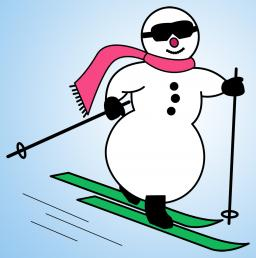We will be pleased if You send us any improvements to this math problem. Thank you!Tips to related online calculators

## Related math problems and questions:

• Ski class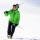Class attend 30 boys and some number of girls. Ski training was attended by 28 boys and all the girls, which was 95% of all students. How many girls attend this class? How many percent is that?
• Sales off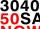Calculate what was the original price of the good, if the price after discount 25% and the subsequent rise of 20% is 400 USD.
• Percent calculationCalculate 51% if 99% is 79.
• Farmer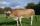The farmer farming 293 ha of arable land and the remaining 20% are meadows and pastures. How much land farmer owns?
• NumberWhat number is 20 % smaller than the number 198?
• Here isHere is a data set (n=117) that has been sorted. 10.4 12.2 14.3 15.3 17.1 17.8 18 18.6 19.1 19.9 19.9 20.3 20.6 20.7 20.7 21.2 21.3 22 22.1 22.3 22.8 23 23 23.1 23.5 24.1 24.1 24.4 24.5 24.8 24.9 25.4 25.4 25.5 25.7 25.9 26 26.1 26.2 26.7 26.8 27.5 27.6 2
• Sum of three numbersThe sum of three numbers from which second number is 20% smaller than the first number and the third number is 25% smaller than the second number is 96. Determine this numbers.
• Children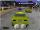Less than 20 children is played various games on the yard. They can create a pairs, triso and quartets. How many children were in the yard when Annie came to them?
• Raspberries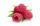Dano had 20 raspberries. John had 90% more raspberries than Dano. Determine how many raspberries have Dano and Juraj together.
• Influenza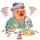For the first week of February by influenza 5% of children in kindergarten. The second week ill 9 next children, bringing the number of patients increased to 20%. How many children went to the kindergarten?
• Price increase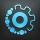A 20 percent price increase meant a 90-crown raise. How many cost a product after?
• Percent chaining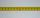Calculate 20% from 70% from 80 km
• Large familyThe average age of all family members (children, mother, father, grandmother, grandfather) is 29 years. The average age of parents is 40 years, grandparents 66 years and all children are 5 years. How many children are there in this family?
• Tennis aces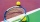The number of aces served by Novak Djokovic in the last 20 tournaments that he has participated in, is shown below. 12 17 13 7 8 14 11 14 10 12 15 9 11 13 6 15 18 5 19 24 1.1 using the raw, determine the range. 1.2 Group the data into a frequency distribu
• Six percents6% of the base is 21. How much is 28% of this base?
• Box and whisker plotConstruct a box and whisker plot for the given data. 56, 32, 54, 32, 23, 67, 23, 45, 12, 32, 34, 24, 36, 47, 19, 43
• Average mark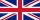Calculate average mark in English, if a student got a couple of 3's, 20% less 2 than 3, and 50% more 1's than the 2's.